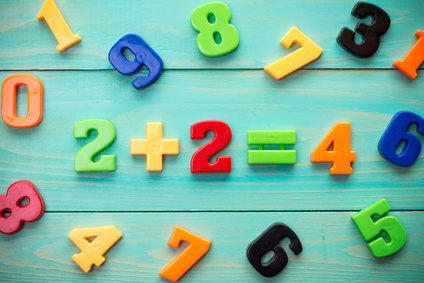# Kindergarten : Free Common Core Math Worksheets

What you will learn: Kindergartners start with numbers, representing and comparing them. We start with taking help from pictures and objects. We progress towards written numerals, modelling simple addition and subtraction, counting, combining, and segregating sets. Get introduced to the base ten system. Compose numbers from 11-19 in tens and ones.

We introduce ourselves to basic geometric shapes like circles, squares, triangles etc. We know about solid shapes like cyclinders, cubes, and spheres. Learn to name 2-d and 3-d shapes. Compose simple shapes to form larger shapes.Not interested in Common Core Standards? Click the button below to the see the traditional curriculum.

Here is the list of all the common core standards for this grade. There are some sample worksheets on the page. Please subscribe to access the whole content in its best form. All of our worksheets are free for non-commercial and personal use. Click on any link to view, print, or download the worksheets.

#### K.CC  :  Counting and Cardinality

K.CC.A Know number names and the count sequence.
K.CC.A.1
Count to 100 by ones and by tens.
K.CC.A.2
Count forward beginning from a given number within the known sequence (instead of having to begin at 1).
K.CC.A.3
Write numbers from 0 to 20. Represent a number of objects with a written numeral 0-20 (with 0 representing a count of no objects).
K.CC.B Count to tell the number of objects.
K.CC.B.4
Understand the relationship between numbers and quantities; connect counting to cardinality.

K.CC.B.4.A
When counting objects, say the number names in the standard order, pairing each object with one and only one number name and each number name with one and only one object.

K.CC.B.4.B
Understand that the last number name said tells the number of objects counted. The number of objects is the same regardless of their arrangement or the order in which they were counted.

K.CC.B.4.C
Understand that each successive number name refers to a quantity that is one larger.
K.CC.B.5
Count to answer "how many?" questions about as many as 20 things arranged in a line, a rectangular array, or a circle, or as many as 10 things in a scattered configuration; given a number from 1-20, count out that many objects.
K.CC.C Compare numbers.
K.CC.C.6
Identify whether the number of objects in one group is greater than, less than, or equal to the number of objects in another group, e.g., by using matching and counting strategies.
K.CC.C.7
Compare two numbers between 1 and 10 presented as written numerals.

#### K.OA  :  Operations and Algebraic Thinking

K.OA.A Understand addition as putting together and adding to, and understand subtraction as taking apart and taking from.
K.OA.A.1
Represent addition and subtraction with objects, fingers, mental images, drawings, sounds (e.g., claps), acting out situations, verbal explanations, expressions, or equations.
K.OA.A.2
Solve addition and subtraction word problems, and add and subtract within 10, e.g., by using objects or drawings to represent the problem.
K.OA.A.3
Decompose numbers less than or equal to 10 into pairs in more than one way, e.g., by using objects or drawings, and record each decomposition by a drawing or equation (e.g., 5 = 2 + 3 and 5 = 4 + 1).
K.OA.A.4
For any number from 1 to 9, find the number that makes 10 when added to the given number, e.g., by using objects or drawings, and record the answer with a drawing or equation.
K.OA.A.5
Fluently add and subtract within 5.

#### K.NBT  :  Number and Operations in Base Ten

K.NBT.A Work with numbers 11-19 to gain foundations for place value.
K.NBT.A.1
Compose and decompose numbers from 11 to 19 into ten ones and some further ones, e.g., by using objects or drawings, and record each composition or decomposition by a drawing or equation (e.g., 18 = 10 + 8); understand that these numbers are composed of ten ones and one, two, three, four, five, six, seven, eight, or nine ones.

#### K.MD  :  Measurement and Data

K.MD.A Describe and compare measurable attributes.
K.MD.A.1
Describe measurable attributes of objects, such as length or weight. Describe several measurable attributes of a single object.
K.MD.A.2
Directly compare two objects with a measurable attribute in common, to see which object has "more of"/"less of" the attribute, and describe the difference. For example, directly compare the heights of two children and describe one child as taller/shorter.
K.MD.B Classify objects and count the number of objects in each category.
K.MD.B.3
Classify objects into given categories; count the numbers of objects in each category and sort the categories by count.

#### K.G  :  Geometry

K.G.A Identify and describe shapes (squares, circles, triangles, rectangles, hexagons, cubes, cones, cylinders, and spheres).
K.G.A.1
Describe objects in the environment using names of shapes, and describe the relative positions of these objects using terms such as above, below, beside, in front of, behind, and next to.
K.G.A.2
Correctly name shapes regardless of their orientations or overall size.
K.G.A.3
Identify shapes as two-dimensional (lying in a plane, "flat") or three-dimensional ("solid").
K.G.B Analyze, compare, create, and compose shapes.
K.G.B.4
Analyze and compare two- and three-dimensional shapes, in different sizes and orientations, using informal language to describe their similarities, differences, parts (e.g., number of sides and vertices/"corners") and other attributes (e.g., having sides of equal length).
K.G.B.5
Model shapes in the world by building shapes from components (e.g., sticks and clay balls) and drawing shapes.
K.G.B.6
Compose simple shapes to form larger shapes.

Worksheet: Kindergarten

Abacus Math

Children will count the beads on the abacus and learn how many more than ten makes that number.

Core Standard: K.CC.B.4

Worksheet: Kindergarten

Subtraction Word Poblems

This a single - digit subtraction word problems worksheet.

Core Standard: K.OA.A.2

Worksheet: Kindergarten

Shapes : Diamond and Oval

Color the diamonds blue, and color the ovals red.

Core Standard: K.G.B.4